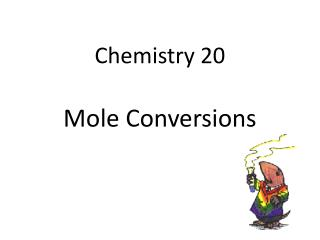Download PresentationChemistry 20 Mole Conversions

# Chemistry 20 Mole Conversions

Download Presentation## Chemistry 20 Mole Conversions

- - - - - - - - - - - - - - - - - - - - - - - - - - - E N D - - - - - - - - - - - - - - - - - - - - - - - - - - -
##### Presentation Transcript

1. Chemistry 20Mole Conversions

2. What is a Mole? • A term of measurement • A counting number • Similar to saying 1 dozen = 12 things • Avogadro's Number (NA) 6.02 x 1023 items A VERY LARGE NUMBER!!! = 1 mole

3. 3.00 moles of little green peas would cover 250 planets the size of Earth 1m deep in green peas • 1.00 mole of basketballs would fill a bag the size of the Earth

4. Mole Conversions Molar Mass Mass In Grams Moles g/mol

5. Molar Mass • Molar mass is the mass of one mole in an element or compound • Atomic mass tells us that the units are grams per mole g/mol Examples: Carbon, C = 12.01 g/mol Phosphorus, P = 30.97 g/mol

6. Molar Mass Examples What is the Molar Mass of: Salt, NaCl: Na = 22.99g/molCl= 35.45g/mol NaCl = 22.99g/mol + 35.45g/mol = 58.44g/mol Glucose, C6H12O6: C6= 6(12.01g/mol) H12 = 12(1.01g/mol) O6 = 6(16.00g/mol) C6H12O6= 6(12.01g/mol) +12(1.01g/mol) 6(16.00g/mol) = 180.18 g/mol

7. Sodium bicarbonate, NaHCO3: Na= 22.99g/mol H=1.01g/mol C=12.01g/mol O = 3(16.00g/mol) NaHCO3= 22.99g/mol + 1.01g/mol + 12.01g/mol + 3(16.00g/mol) = 84.01g/mol

8. Mole Conversion Examples A grade eleven chemistry student has 3.00 g of boron tribromide (BBr3). How many moles of BBr3 is this? Molar mass of BBr3 = 10.81 + 3(79.90) = 250.51g/mol 3.00g BBr3 x1mole = 0.0120 mole BBr3 250.51g REMEMBER SIG FIGS!

9. If I have 50.0g of calcium chlorate (CaClO3)2 how many moles do I have? Molar Mass (CaClO3)2 = 2(40.08) + 2(35.45) + 6(16.00) = 247.06 g/mol 50.0g (CaClO3)2 x 1 mole = 0.202 mole (CaClO3)2 247.06g Sig Figs

10. Determine the mass of the following amount: 6.94 mol barium chloride (BaCl2) Molar Mass BaCl2 = 137.33 + 2(35.45) = 208.23 g/mol 6.94 molBaCl2 x 208.23 g = 1.45 x 103 g BaCl2 1 mole Remember only 3 sig figs

11. Chemistry 20Mole Conversions

12. Mole Conversions Avogadro’s Number Respective Particles Moles 6.02 x 1023 molecules or atoms or formula units

13. Mole Conversion Examples If a chemist is experimenting with 5 700 000 000 atoms of lead how many moles is she dealing with? Avogadro’s number = 6.02 x 1023 atoms 5 700 000 000 atoms Pb x 1 mole = 9.5 x 10-15mol 6.02 x 1023 atoms 2 sig figs 2 sig figs

14. How many moles is in 2.97 x 1025 atoms of Vanadium? 2.97 x 1025 atoms of V x 1 mole 6.02 x 1023 atoms = 49.3 moles of V

15. Determine the number of molecules in the following: • 1.01 moles of bismuth 1.01 mol Bi x 6.02 x 1023 atoms 1 mole = 6.08 x 1023atoms of Bi

16. b) 2.5 moles of manganese 2.5 molMnx 6.02 x 1023atoms 1 mole = 1.5 x 1024atoms of Mn

17. If the students in a chemistry 20 class have 7.66 x 1019 molecules of hydrogen peroxide, H2O2, how many moles do they have? 7.66 x 1019 molecules x 1 mole 6.02 x 1023 molecules = 1.27 x 10-4 molecules of H2O2

18. Chemistry 20Mole Conversions

19. Mole Conversions Litres of Gas at STP Volume Moles 22.4 L

20. Mole Conversion Examples Determine the volume, in liters, occupied by 0.030 moles of helium gas at STP. 0.030 moles He(g) x 22.4L = 0.67 L of He(g) 1 mole REMEMBER UNITS

21. What is the volume of 0.05 mol of neon gas at STP? 0.05 mol Ne x 22.4L = 1.12L of Ne(g) 1 mole

22. How many moles of argon atoms are present in 11.2 L of argon gas at STP? 11.2L Ar(g) x 1 mole = 0.5 mole Ar(g) 22.4L

23. What is the volume of 1.2 moles of water vapor at STP? 1.2 mol H2O(g) x 22.4L = 26.88L of H2O 1 mole

24. Chemistry 20Mole Conversions

25. Mole Conversions Representative Particles Volume at STP x 6.02 x 1023particles 1 mole X 22.4L 1 mole x 1 mole 6.02 x 1023 particles x 1 mole 22.4L MOLE x 1mole molar mass x molar mass 1mole Mass

26. Mixed Mole Conversion • Write down the question’s the given and the unknown. • Convert the given unit to number of moles. • Convert the number of moles found to the unknown unit. • Make sure the answer has the correct unit and number of sig figs.

27. Mole Conversion Examples Calculate the mass, in grams (g), of the following: • 3.00L of O2(g) 3.00L of O2(g) x 1 mole x 32.00 g = 4.29 g O2(g) 22.4L 1mole b) 15 atoms of gold 15 atoms Au(s) x 1 mole x 196.97g = 4.9 x 10-21 g Au 6.02 x 1023 atoms 1 mole

28. Calculate the number of atoms in 2.0L of SO3 gas. 2.0LSO3(g)x 1 mole x 6.02 x 1023 atoms = 5.3 x 1022 atoms SO3 22.4 L 1 mole

29. If the students in a Chemistry 20 class have 3.25 x 105 molecules of CO2(g), how many litres do they have? 3.25 x 105molecules of CO2(g) x 1 mole = 5.40 x 10-29 mole 6.02 x 1023molecules 5.40 x 10-29mole CO2 x 22.4L = 1.21 x 10-17 L CO2 1 mole